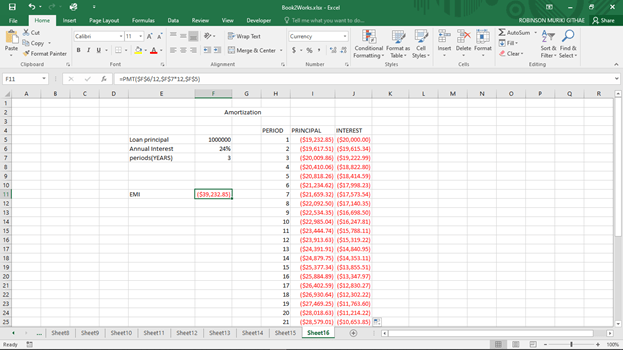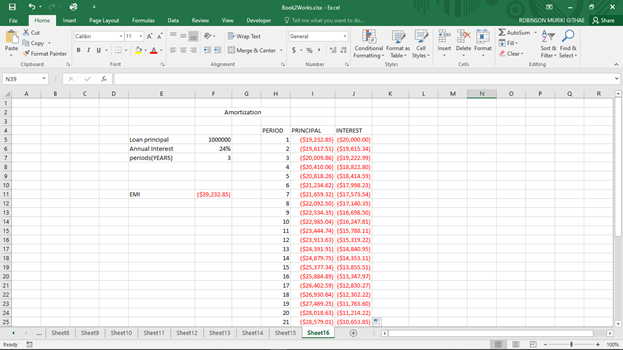# Excel formula for Amortization

The process of amortization involves paying back a loan for a given period of time until the loan is fully paid. For a long time, amortization calculation used to be done using a pen, paper and calculator but things are now changing. We can calculate loan amortization in Excel using formulas. Some of the basics needed to calculate amortization include knowledge on how to use: NPER, PV, RATE, PMT, PPMT, IPMT

1. Finding EMI using PMT

EMI (equated monthly installment) is the monthly amount paid by the loaned (principal+ interest) and is calculated using the PMT () function. To find the EMI we supply the PMT function with the appropriate parameters.

The general syntax of PMT function in Excel is:

=PMT (Rate, Nper, -PV)Note: the PMT function should always return a negative value by default hence we include the PV as negative. The reason why it returns a negative is that it is an expectation that this is money that flows away from the loaned.

Rate: this is the interest rate (for each payment period) provided by the loan.

Nper: Total Number of periods one is expected to repay the loan (usually in months in most cases)

Pv: Represents the present value of the loan(principal) to be repaid by the loaned.

PMT function is used to return the sum of interest and principal for each payment period. It can thus be used to define the monthly payment amount of a loan if the interest rate is constant.

2. NPER

The NPER function aids us to know the number of periods taken to repay. The general syntax of the formula is:

=NPER (Rate, PMT, PV)

3. Calculating interests

It is important to note that for most loans the interest charges are higher at the beginning but reduce as more payments are done.Finding interest included in EMI

Interest =EMI- principal

4. Alternatively

Interest=IPMT (rate, per, nper, pv)The entire figures for interest should be as follows:Finding principal included in the EMI

Principal=PPMT (Rate, per, NPER, PV)

per: represents the number of periods that have already been paid while including the period you wish to calculate the EMI principal

Nper: represents the total number of periods the loan is to be paid.

PV: is the loan amount/present value

Ensure that the periods reach 36.After calculation of principal, it should be something similar to:5. Calculating PV

=PV (rate, nper, pmt)

6. Finding current loan balance

Current loan Balance= previous balance -principal